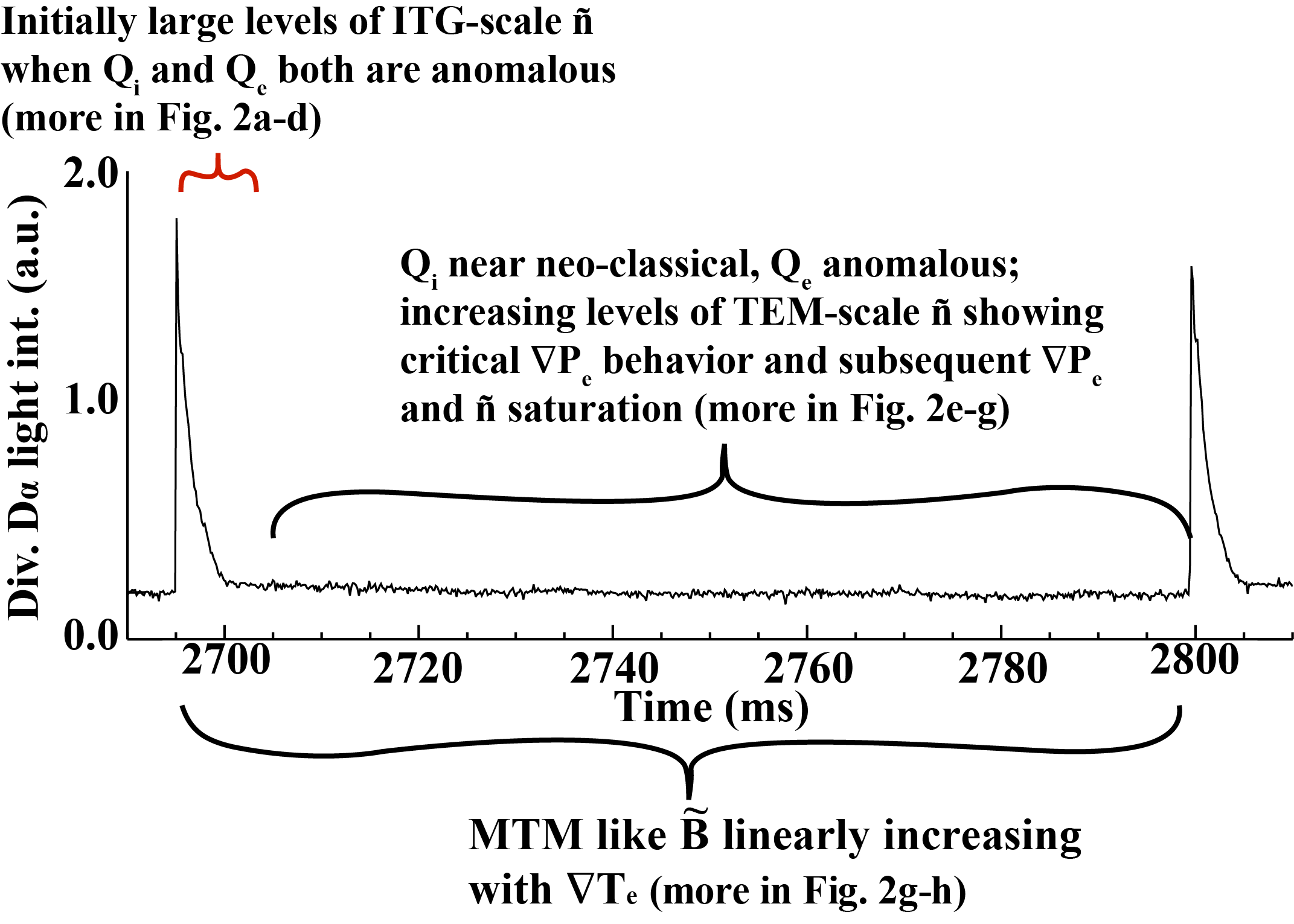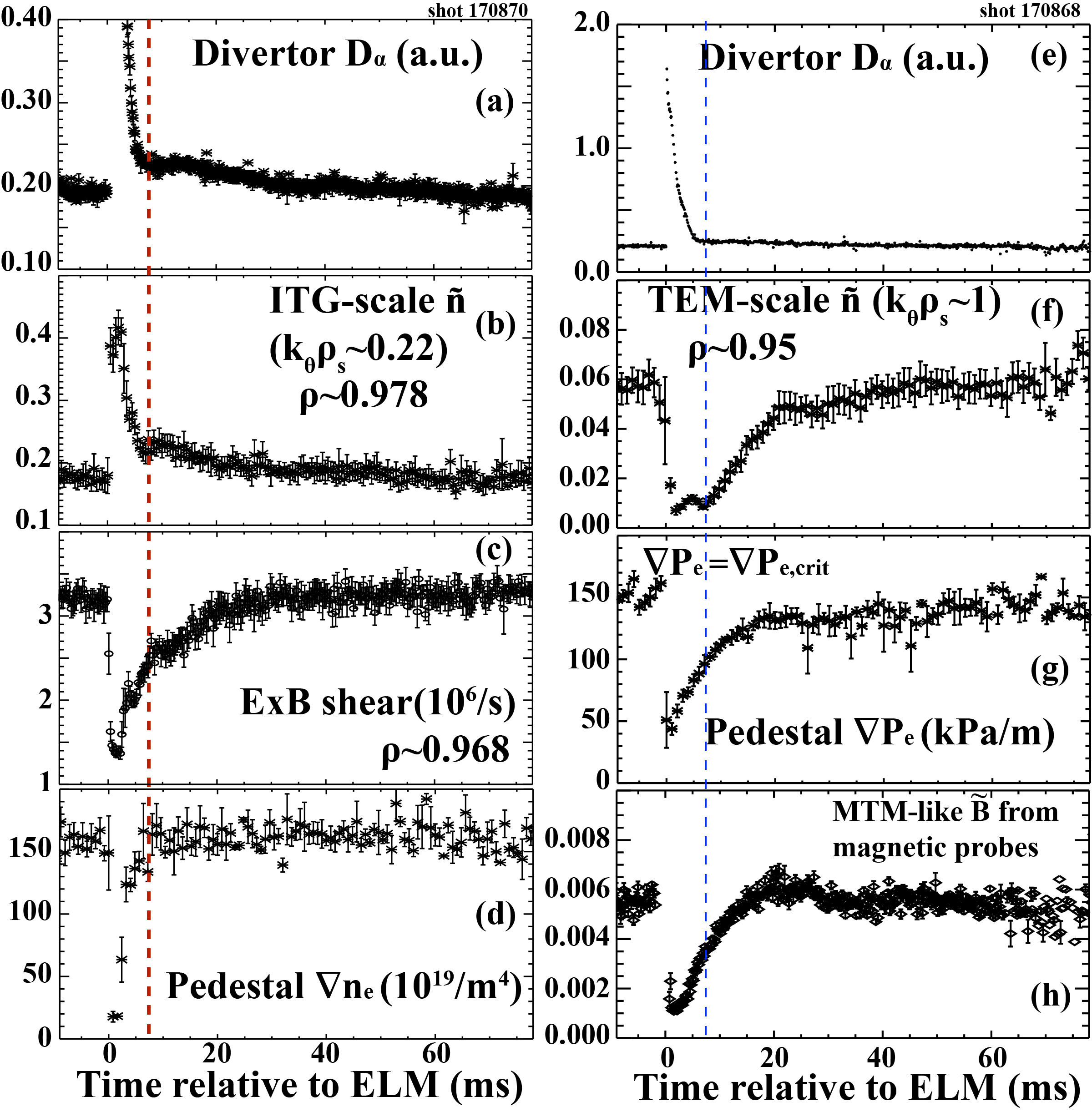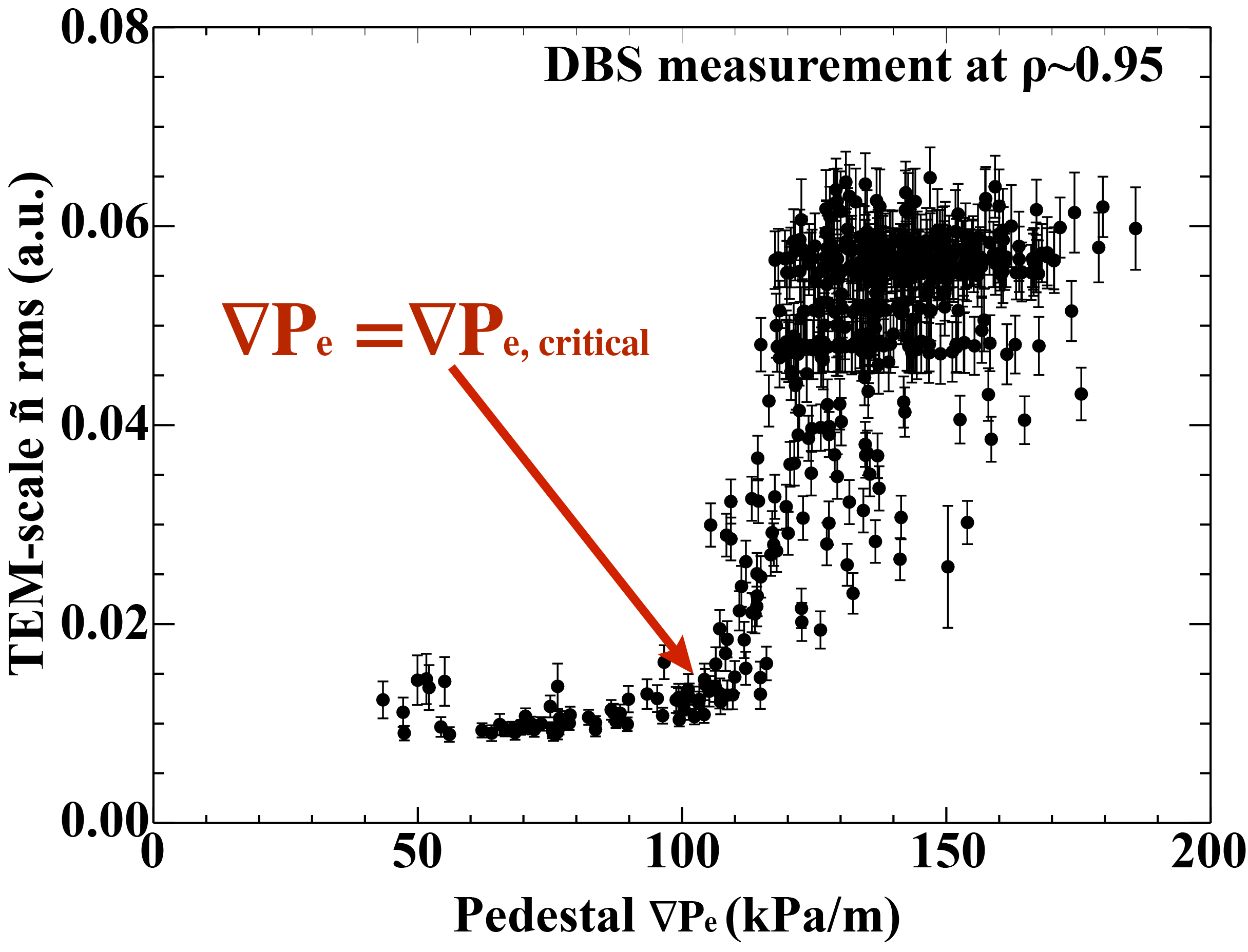Since 18 of December 2019 conferences.iaea.org uses Nucleus credentials. Visit our help pages for information on how to Register and Sign-in using Nucleus.

# 28th IAEA Fusion Energy Conference (FEC 2020)

May 10 – 15, 2021
Virtual Event
Europe/Vienna timezone
The Conference will be held virtually from 10-15 May 2021

## [REGULAR POSTER TWIN] New understanding of multi-scale/multi-field pedestal turbulence, transport, and gradient behavior during type-I ELMs on the DIII-D tokamak

May 11, 2021, 2:00 PM
4h 45m
Virtual Event

#### Virtual Event

Regular Poster Magnetic Fusion Experiments

### Speaker

Kshitish Kumar Barada (University of California-Los Angeles)

### Description

Detailed new H-mode pedestal measurements in the inter-ELM periods of DIII-D discharges find that ion temperature gradient (ITG)-scale density fluctuations (ñ) can explain anomalous ion heat flux (Q$_{i}$) during the ELM (Fig. 1), followed by Q$_{i}$ becoming neo-classical$^{1}$ until the next ELM, and with trapped electron mode (TEM) and microtearing mode (MTM) like ñ and magnetic fluctuations ($\tilde{B}$) able to explain anomalous electron heat flux (Q$_{e}$) between ELMs (Fig. 1). The observed ITG-scale, TEM-scale, and MTM-like turbulence (ñ, $\tilde{B}$) behaviors are in clear contrast to the often assumed suppression or near suppression of these modes in the pedestal during inter-ELM periods$^{2}$. This new information impacts our fusion power goals as it will lead to improved predictive capability when existing (and new) models take into account the transport caused by these modes and their various temporal phases during the inter-ELM period. The goal of predicting and controlling pedestal pressure evolution and improving ELM control (or elimination) is believed necessary to optimize fusion performance and to protect plasma facing components of ITER and future burning plasmas.Inter-ELM behavior of electron pedestal density, temperature, and pressure gradients ( ∇n$_{e,ped}$, ∇T$_{e,ped}$,and ∇P$_{e,ped}$) estimated from Thomson scattering measurements and pedestal localized turbulence were studied in ITER similar shape (ISS) H-mode plasmas. The gradients of electron temperature, density, and pressure remain approximately saturated for more than 75% of the inter-ELM period (Fig 2). Immediately after an ELM, evolution of ITG-scale low-k (k$_{\theta}\rho_{s}$~0.2, k$_{\theta}$ is the fluctuation poloidal wavenumber and $\rho_{s}$ is the ion Larmor radius) ñ (Fig 2b), measured by Doppler backscattering at the foot of the pedestal, correlates strongly with a rapid increase and subsequent saturation of ∇n$_{e,ped}$ (Fig 2d). This low-k ñ increases strongly when the pedestal profiles relax after an ELM crash, is correlated with a decrease in local E$\times$B shear and later is suppressed by increase in local E$\times$B shear (Fig 2c). The evolution of this ITG-scale ñ amplitude correlates well with the divertor D$_{\alpha}$ light intensity (Fig 2a) and ∇T$_{e,ped}$ (not shown) starts to increase after suppression of the ITG-scale ñ. These observations are consistent with ITG-scale ñ driving electron and ion heat flux as well as particle flux just after the ELM crash. Experimental results from ASDEX-U indicating the anomalous nature of both ion and electron heat fluxes right after an ELM crash, are consistent with our observations$^{1}$.
TEM-scale intermediate-k (k$_{\theta}\rho_{s}$~1) ñ measured by Doppler backscattering in the steep gradient region of the pedestal shows a critical ∇P$_{e,ped}$ behavior with ñ increasing rapidly when a critical ∇P$_{e,ped}$ is reached (Fig. 3). As the pressure gradient increases, increases in E$\times$B velocity shear (not shown here) leads to saturation of TEM-scale ñ (at ~120 kPa/m, Fig 3) indicating a balance between saturated values of gradient drive, shear suppression, and turbulence level. The approximate saturation of ∇P$_{e,ped}$ that follows the large increase of TEM-scale ñ at critical ∇P$_{e,ped}$ also suggests the related turbulence transport has a role in clamping ∇P$_{e,ped}$. Note however, although ∇P$_{e,ped}$ becomes approximately constant within 25% of the ELM cycle there remains a slow increase in ∇P$_{e,ped}$ as shown in Fig 2g and Fig 3. Magnetic turbulence $\tilde{B}$, measured by polarimetry and fast magnetic probes (Fig 2h), has been identified as MTM turbulence $^{3}$ and increases with ∇T$_{e,ped}$ (∇T$_{e,ped}$ not shown but is very similar to the ∇P$_{e,ped}$ shown in Fig. 2g ) in the inter-ELM period. Both TEM-scale ñ and MTM-like $\tilde{B}$ can cause significant electron thermal transport and may explain the anomalous nature of Q$_{e}$ in the pedestal as observed by transport calculations using experimental profiles (in ASDEX-U$^{1}$ and DIII-D$^{4}$ tokamaks).Initial linear trapped-gyro-Landau-fluid (TGLF)$^{5}$ calculations in the nearly saturated phase of the ELM cycle (60-99% of the ELM cycle, refer Fig 2) show that intermediate-k (k$_{\theta}\rho_{s}$~1) electrostatic fluctuations are the most unstable modes in the steep gradient region of the pedestal and propagate in the electron diamagnetic direction in the plasma frame. The growth rates are approximately 1.5-2 times more than the local E$\times$B shearing rates and so are relatively unaffected by the shearing. This is qualitatively consistent with the observation of these modes by the DBS system. These modes are still the most unstable modes when electromagnetic effects are included in TGLF calculations. Interestingly, scans of electron temperature and density scale lengths indicate that these modes are driven by both ∇T$_{e}$ and ∇n$_{e}$ in qualitative agreement with the observed ñ vs ∇P$_{e}$ behavior (Fig 2 and 3). Further, the TGLF simulations indicate a critical gradient type response similar to experiment (Fig 3). Based on these above TGLF results we initially identify these modes as TEM type instabilities. The above TGLF results are consistent with TEM type instabilities leading us to initially identify the experimentally observed density fluctuations to be trapped electron modes.
The new scientific understanding reported here on the ELM and inter-ELM turbulence, thermal fluxes, and temporal behavior are critical to testing, validating, and improving current models and simulations. These improved models and simulations are in turn fundamental to predicting and controlling pedestal pressure and ELMs (or even ELM elimination) necessary for optimizing and protection of future fusion devices.

This work was supported by the US Department of Energy under grants DE-FG02-08ER54984, DE-AC02-09CH11466, DE-FG02-08ER54999, DE-SC0019302 and DE-FC02-04ER54698.

$^{1}$E. Viezzer et al., Nucl. Fusion 57, 022020 (2017).
$^{2}$P.B. Snyder et al., Nuclear Fusion 51, 103016 (2011).
$^{3}$J. Chen et al., Submitted to IAEA FEC 2020.
$^{4}$S. Haskey et al., Submitted to IAEA FEC 2020.
$^{5}$G. Staebler et al., Phys. Plasmas 14, 055909 (2007).

Country or International Organization United States University of California-Los Angeles

### Primary author

Kshitish Kumar Barada (University of California-Los Angeles)

### Co-authors

Terry Rhodes (UCLA) Shaun Haskey (Princeton Plasma Physics Laboratory) R.J. Groebner (General Atomics) Dr Santanu Banerjee (College of William and Mary) Ahmed Diallo (PPPL) Florian M. Laggner (Princeton University) Zheng Yan (University of Wisconsin-Madison) Dr Lei Zeng (University of California, Los Angeles) Dr Guiding Wang (University of California, Los Angeles)